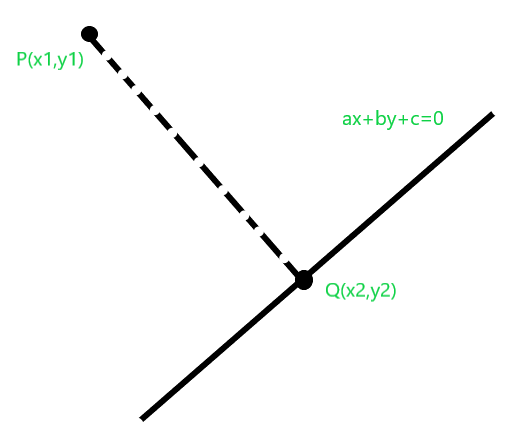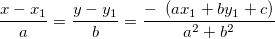# Find foot of perpendicular from a point in 2 D plane to a Line

Given a point P in 2-D plane and equation of a line, the task is to find the foot of the perpendicular from P to the line.

Note: Equation of line is in form ax+by+c=0.

Examples:

```Input :  P=(1, 0), a = -1, b = 1, c = 0
Output : Q = (0.5, 0.5)
The foot of perpendicular from point (1, 0)
to line -x + y = 0 is (0.5, 0.5)

Input :  P=(3, 3), a = 0, b = 1, c = -2
Output : Q = (3, 2)
The foot of perpendicular from point (3, 3)
to line y-2 = 0 is (3, 2)
```

## Recommended: Please try your approach on {IDE} first, before moving on to the solution.Since equation of the line is given to be of the form ax + by + c = 0. Equation of line passing through P and is perpendicular to line. Therefore equation of line passing through P and Q becomes ay – bx + d = 0. Also P passes through line passing through P and Q, so we put coordinate of P in the above equation:

```ay1 - bx1 + d = 0
or, d = bx1 - ay1
```

Also, Q is the intersection of the given line and the line passing through P and Q. So we can find the solution of:

```ax + by + c = 0
and,
ay - bx + (bx1-ay1) = 0
```

Since a, b, c, d all are known we can find x and y here as:Below is the implementataion of the above approach:

## C++

 `// C++ program for implementation of ` `// the above approach ` `#include ` `using` `namespace` `std; ` ` `  `// Function to find foot of perpendicular from ` `// a point in 2 D plane to a Line ` `pair<``double``, ``double``> findFoot(``double` `a, ``double` `b, ``double` `c, ` `                              ``double` `x1, ``double` `y1) ` `{ ` `    ``double` `temp = -1 * (a * x1 + b * y1 + c) / (a * a + b * b); ` `    ``double` `x = temp * a + x1; ` `    ``double` `y = temp * b + y1; ` `    ``return` `make_pair(x, y); ` `} ` ` `  `// Driver Code ` `int` `main() ` `{ ` `    ``// Equation of line is ` `    ``// ax + by + c = 0 ` `    ``double` `a = 0.0; ` `    ``double` `b = 1.0; ` `    ``double` `c = -2; ` ` `  `    ``// Coordinates of point p(x1, y1). ` `    ``double` `x1 = 3.0; ` `    ``double` `y1 = 3.0; ` ` `  `    ``pair<``double``, ``double``> foot = findFoot(a, b, c, x1, y1); ` `    ``cout << foot.first << ``" "` `<< foot.second; ` ` `  `    ``return` `0; ` `} `

## Java

 `import` `javafx.util.Pair; ` ` `  `// Java program for implementation of ` `// the above approach ` `class` `GFG  ` `{ ` ` `  `// Function to find foot of perpendicular from ` `// a point in 2 D plane to a Line ` `static` `Pair findFoot(``double` `a, ``double` `b, ``double` `c, ` `                            ``double` `x1, ``double` `y1) ` `{ ` `    ``double` `temp = -``1` `* (a * x1 + b * y1 + c) / (a * a + b * b); ` `    ``double` `x = temp * a + x1; ` `    ``double` `y = temp * b + y1; ` `    ``return` `new` `Pair(x, y); ` `} ` ` `  `// Driver Code ` `public` `static` `void` `main(String[] args)  ` `{ ` `    ``// Equation of line is ` `    ``// ax + by + c = 0 ` `    ``double` `a = ``0.0``; ` `    ``double` `b = ``1.0``; ` `    ``double` `c = -``2``; ` ` `  `    ``// Coordinates of point p(x1, y1). ` `    ``double` `x1 = ``3.0``; ` `    ``double` `y1 = ``3.0``; ` ` `  `    ``Pair foot = findFoot(a, b, c, x1, y1); ` `    ``System.out.println(foot.getKey() + ``" "` `+ foot.getValue()); ` `    ``} ` `} ` ` `  `// This code contributed by Rajput-Ji `

## Python3

 `# Python3 implementation of the approach  ` ` `  `# Function to find foot of perpendicular  ` `# from a point in 2 D plane to a Line  ` `def` `findFoot(a, b, c, x1, y1):  ` ` `  `    ``temp ``=` `(``-``1` `*` `(a ``*` `x1 ``+` `b ``*` `y1 ``+` `c) ``/``/` `                  ``(a ``*` `a ``+` `b ``*` `b))  ` `    ``x ``=` `temp ``*` `a ``+` `x1  ` `    ``y ``=` `temp ``*` `b ``+` `y1  ` `    ``return` `(x, y)  ` ` `  `# Driver Code  ` `if` `__name__ ``=``=` `"__main__"``: ` ` `  `    ``# Equation of line is  ` `    ``# ax + by + c = 0  ` `    ``a, b, c ``=` `0.0``, ``1.0``, ``-``2` `     `  `    ``# Coordinates of point p(x1, y1).  ` `    ``x1, y1 ``=` `3.0``, ``3.0` ` `  `    ``foot ``=` `findFoot(a, b, c, x1, y1)  ` `    ``print``(``int``(foot[``0``]), ``int``(foot[``1``]))  ` `         `  `# This code is contributed ` `# by Rituraj Jain `

## C#

 `// C# program for implementation of  ` `// the above approach  ` `using` `System; ` ` `  `class` `GFG  ` `{  ` `    ``// Pair class ` `    ``public` `class` `Pair ` `    ``{ ` `        ``public` `double` `first,second; ` `        ``public` `Pair(``double` `a,``double` `b) ` `        ``{ ` `            ``first = a; ` `            ``second = b; ` `        ``} ` `    ``} ` ` `  `// Function to find foot of perpendicular from  ` `// a point in 2 D plane to a Line  ` `static` `Pair findFoot(``double` `a, ``double` `b, ``double` `c,  ` `                            ``double` `x1, ``double` `y1)  ` `{  ` `    ``double` `temp = -1 * (a * x1 + b * y1 + c) / (a * a + b * b);  ` `    ``double` `x = temp * a + x1;  ` `    ``double` `y = temp * b + y1;  ` `    ``return` `new` `Pair(x, y);  ` `}  ` ` `  `// Driver Code  ` `public` `static` `void` `Main(String []args)  ` `{  ` `    ``// Equation of line is  ` `    ``// ax + by + c = 0  ` `    ``double` `a = 0.0;  ` `    ``double` `b = 1.0;  ` `    ``double` `c = -2;  ` ` `  `    ``// Coordinates of point p(x1, y1).  ` `    ``double` `x1 = 3.0;  ` `    ``double` `y1 = 3.0;  ` ` `  `    ``Pair foot = findFoot(a, b, c, x1, y1);  ` `    ``Console.WriteLine(foot.first + ``" "` `+ foot.second);  ` `    ``}  ` `}  ` ` `  `// This code contributed by Arnab Kundu `

## PHP

 ` `

Output:

```3 2
```

Attention reader! Don’t stop learning now. Get hold of all the important DSA concepts with the DSA Self Paced Course at a student-friendly price and become industry ready.

My Personal Notes arrow_drop_upAlways try to improve and willing to learn

If you like GeeksforGeeks and would like to contribute, you can also write an article using contribute.geeksforgeeks.org or mail your article to contribute@geeksforgeeks.org. See your article appearing on the GeeksforGeeks main page and help other Geeks.

Please Improve this article if you find anything incorrect by clicking on the "Improve Article" button below.